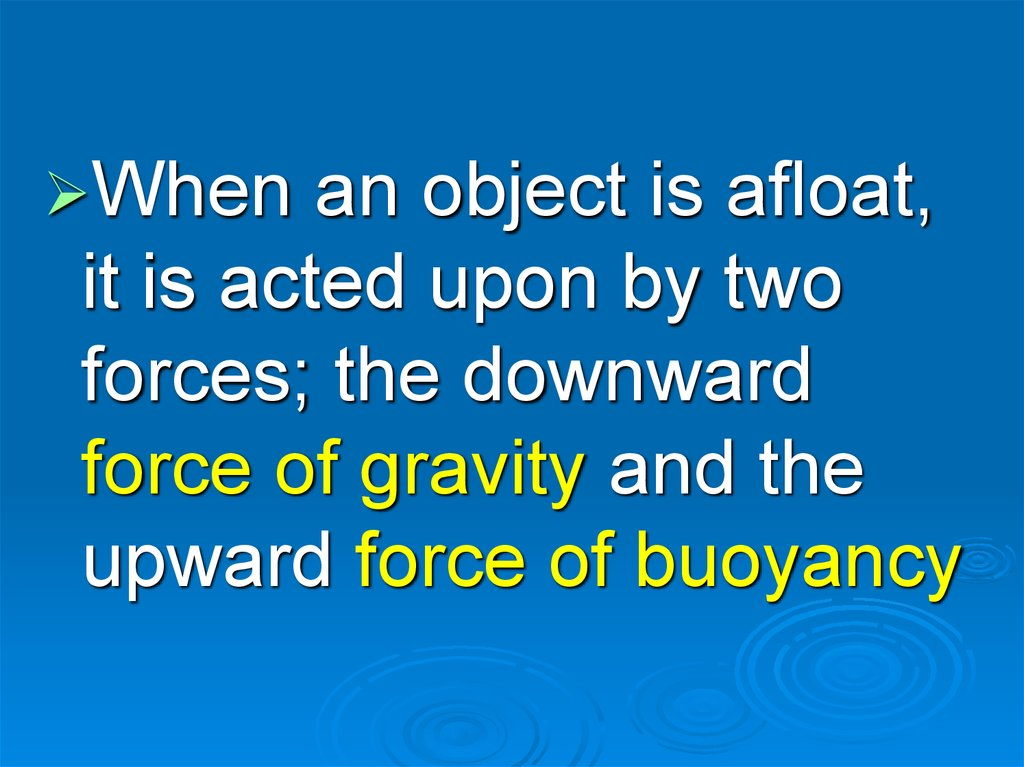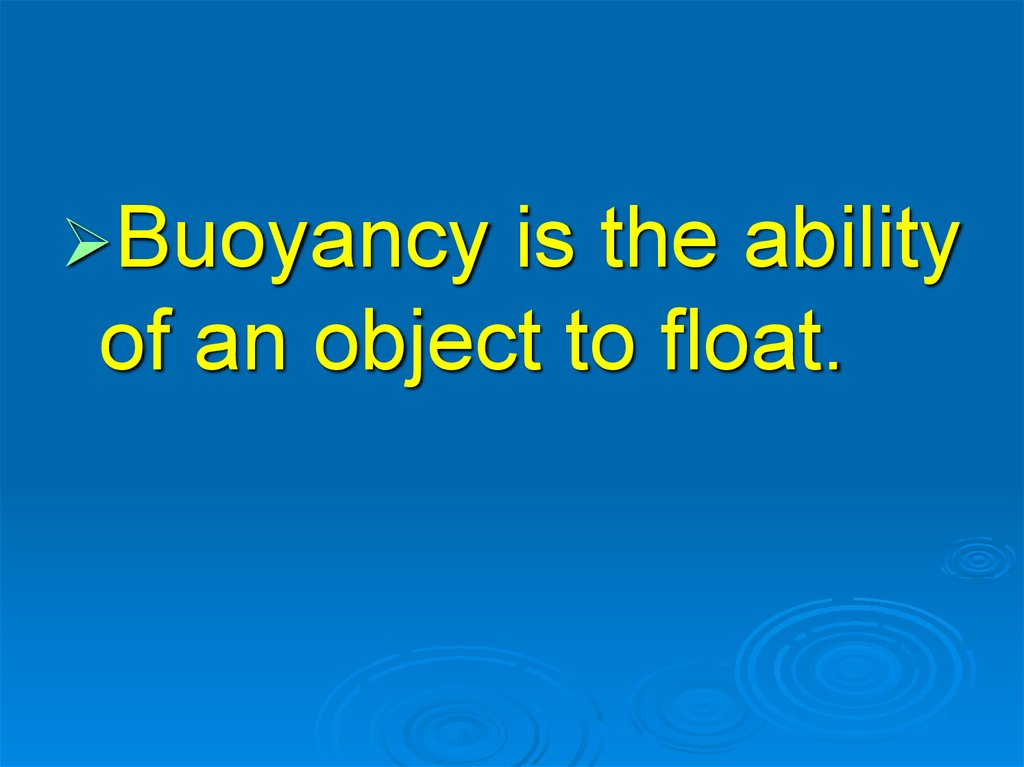# Ship’s stability

SHIP’S
STABILITY

## 2. Slayt 2

When
an object is afloat,
it is acted upon by two
forces; the downward
force of gravity and the
upward force of buoyancy

## 3. Slayt 3

Buoyancy
is the ability
of an object to float.

## 4. Slayt 4

If an object is placed into water
and the weight of this object is
greater than the weight of an
equal volume of water, the
object will sink. It sinks
because the force of buoyancy
is less than the weight of the
object.

## 5. Slayt 5

However, if the weight of the
object is less than the
weight of an equal volume
of water, the object will float

## 6. Slayt 6

Centre
of gravity is the point of
application of the gravitational
force on the ship.

## 7. Slayt 7

Centre
of buoyancy is the
point of application of the
buoyancy force on a ship.

## 9. Slayt 9

When
side of a ship, there will be a
temporary shift in the centre of
gravity. Once the ship tilts to
one side, the centre of
buoyancy will also shift.

## 10. Slayt 10

The
ship's centre of gravity
determines the ship's stability.

## 11. Slayt 11

Stability may be defined as
the ability of a vessel to
after inclination.

G-GRAVITY
M-METACENTRE

M
G
L
B'

G
M
W
L
B'

G
M
W
L
B"

## 16. State that the following sentences true or false according to the text.

1-Two
forces act upon the
object when it is afloat.

## 17. Slayt 17

2-The
centre of gravitational
force is centre of buoyancy.

## 18. Slayt 18

3-If ship inclines, the centre
of buoyancy changes.

## 19. Slayt 19

4-If M is below G, this means
that the ship is not stable.

## 20. SOME WORDS

Deflection (n) - Deviation,
being deflected

## 21. Slayt 21

Equilibrium (n) - Balance

## 22. Slayt 22

Incline (v) - To deviate from a
vertical position, to lean, to
slope

## 23. Slayt 23

Shift (v) - To change position or
direction

## 24. Slayt 24

Sink (v) - To fall down below the
surface of the water, to submerge

## 26. Slayt 26

Tilt (v) - To incline, to slope

## 27. B-Underline the true word or phrase in italics

1-The
gravitational force is
upward / downward force.

## 28. Slayt 28

2-If
metacentre is above / below
centre of gravity , this ship will be
stable.

## 29. Slayt 29

3-When
the force of buoyancy is
less than the weight of the object,
it sinks / floats.